# Electronics and Communication Engineering - Electromagnetic Field Theory

36.
In order to receive a vertically polarized wave, the conductor of the dipole should be mounted
horizontally
vertically
at an angle of 45°
none of the above
Explanation:
No answer description is available. Let's discuss.

37.
Which of the following statements is incorrect?
The line integral of B around the circuit is called magnetomotive force
Normal components of B are continuous but the normal components of H are not continuous
Magnetic flux lines are bent away from the normal in the medium with the highest value of permeability
The unit of permeability is henry/metre
Explanation:
No answer description is available. Let's discuss.

38.
Reflectors and lens antennas are commonly used
in LF communication systems
not beyond 100 kHz
in the range 10 MHz to 100 MHz
above 100 MHz
Explanation:
No answer description is available. Let's discuss.

39.
In case of concentric conductors of radius a and b the value of capacitance (C) is given by
C = 0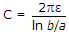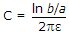none of the above
-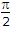radius
-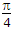radius
-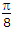radius